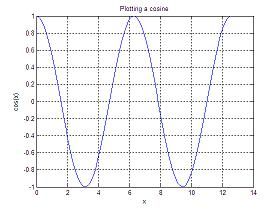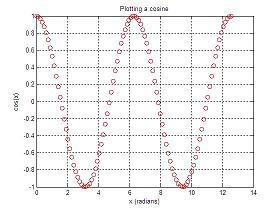Examples: MATLAB Plots

 In this group of examples, we are going to create several MATLAB plots. We are going to produce some figures with simple scripts to show first a small number of points, and we'll show then more points to see how this affect the general image. 2D Cosine Plot y=cos(x), forYou can type this in an 'm-file':

% Simple script to plot a cosine
% vector x takes only 10 values
x = linspace(0, 2*pi, 10);
y = cos(x);
plot(x,y)
xlabel('x')
ylabel('cos(x)')
title('Plotting a cosine')
grid on

The result is:If we use 100 points rather than 10, and evaluate two cycles instead of one (like this):

x = linspace(0, 4*pi, 100);

We obtain a different curve:Now, a variation with line-syles and colors (type 'help plot' to see the options for line-types and colors):

clear; clc; close all;
% Simple script to plot a cosine
% vector x takes only 10 values
x = linspace(0, 4*pi, 100);
y = cos(x);
plot(x,y, 'ro')
ylabel('cos(x)')
title('Plotting a cosine')
grid onSpace curve

Use the command plot3(x,y,z) to plot the helix:

If  x(t)=sin(t), y(t)=cos(t), z(t)=(t) for every t in,
then, you can type this code in an 'm-file' or in the command window:

% Another way to assign values to a vector
t = 0 : pi/50 : 10*pi;
plot3(sin(t),cos(t),t);You can use the 'help plot3' command to find out more details.

From 'Matlab Plots' to home

From 'Matlab Plots' to 'Matlab Examples'

 Top Functions 2D Drawings 3D Graphics